# How to Calculate and Solve for Frenkel Defect | Ceramics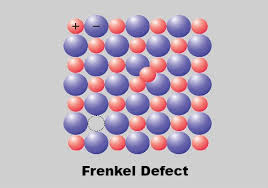The image above represents frenkel defect.

To compute for frenkel defect, four essential parameters are needed and these parameters are N, activation energy (Qfr), Boltzmann’s Constant (K) and temperature (T).

The formula for calculating the frenkel defect:

Nfr = N exp (-Qfr / 2KT)

Where:

Nfr = Frenkel Defect
Qfr = Activation Energy
K = Boltzmann’s Constant
T = Temperature

Let’s solve an example;
Find the frenkel defect when the activation energy is 34, N is 22, Temperature is 12 and the boltzmann’s constant is 1.38064852e-23.

This implies that;

Qfr = Activation Energy
K = Boltzmann’s Constant
T = Temperature

Nfr = N exp (-Qfr / 2KT)
Nfr = (22)exp(-(34) / 2(1.38064852e-23)(14))
Nfr = (22)exp((-34) / (3.865815856e-22))
Nfr = (22)exp(-8.795038684325786e+22)
Nfr = (22)(0)
Nfr = 0

Therefore, the frenkel defect is 0.

Calculating the Activation Energy when the Frenkel Defect, the Boltzmann’s Constant and the Temperature is Given.

Qfr = – (In (Nfr / N) x 2KT)

Where;

Qfr = Activation Energy
Nfr = Frenkel Defect
K = Boltzmann’s Constant
T = Temperature

Let’s solve an example;
Given that the frenkel defect is 20, the boltzmann’s constant is 5, the temperature is 2 and N is 10. Find the activation energy?

This implies that;

Nfr = Frenkel Defect = 20
K = Boltzmann’s Constant = 5
T = Temperature = 2
N = 10

Qfr = – (In (Nfr / N) x 2KT)
Qfr = – (In (20 / 10) x 2 x 5 x 2)
Qfr = – (In 2 x 20)
Qfr = – (In 40)
Qfr = – 3.688

Therefore, the activation energy is – 3.688.

Calculating the Boltzmann’s Constant when the Frenkel Defect, the Activation Energy and the Temperature is Given.

K = – Qfr / In (Nfr / N) x 1 / 2T

Where;

K = Boltzmann’s Constant
T = Temperature
Qfr = Activation Energy
Nfr = Frenkel Defect

Let’s solve an example;
Find the boltzmann’s constant when the temperature is 20, the frenkel defect is 30, the activation energy is 12 and N is 6.

This implies that;

T = Temperature = 20
Qfr = Activation Energy = 12
Nfr = Frenkel Defect = 30
N = 6

K = – Qfr / In (Nfr / N) x 1 / 2T
K = – 12 / In (30 / 6) x 1 / 2 x 20
K = – 12 / In 5 x 1 / 40
K = – 12 / 1.609 x 0.025
K = – 7.458 x 0.025
K = – 0.1864

Therefore, the boltzmann’s constant is – 0.1864

Calculating the Temperature when the Frenkel Defect, the Activation Energy and the Boltzmann’s Constant is Given.

T = – Qfr / In (Nfr / N) x 1 / 2K

Where;

T = Temperature
Qfr = Activation Energy
Nfr = Frenkel Defect
K = Boltzmann’s Constant

Let’s solve an example;
Find the temperature when the activation energy is 40, the frenkel defect is 60, the boltzmann’s constant is 20, N is 30.

This implies that;

Qfr = Activation Energy = 40
Nfr = Frenkel Defect = 60
K = Boltzmann’s Constant = 20
N = 30

T = – Qfr / In (Nfr / N) x 1 / 2K
T = – 40 / In (60 / 30) x 1 / 2 x 20
T = – 40 / In 30 x 1 / 40
T = – 40 / 3.401 x 0.025
T = – 11.761 x 0.025
T = – 0.294

Therefore, the temperature is – 0.294.

Nickzom Calculator – The Calculator Encyclopedia is capable of calculating the frenkel defect.

To get the answer and workings of the frenkel defect using the Nickzom Calculator – The Calculator Encyclopedia. First, you need to obtain the app.

You can get this app via any of these means:

To get access to the professional version via web, you need to register and subscribe for NGN 1,500 per annum to have utter access to all functionalities.
You can also try the demo version via https://www.nickzom.org/calculator

Apple (Paid) – https://itunes.apple.com/us/app/nickzom-calculator/id1331162702?mt=8
Once, you have obtained the calculator encyclopedia app, proceed to the Calculator Map, then click on Materials & Metallurgical under Engineering.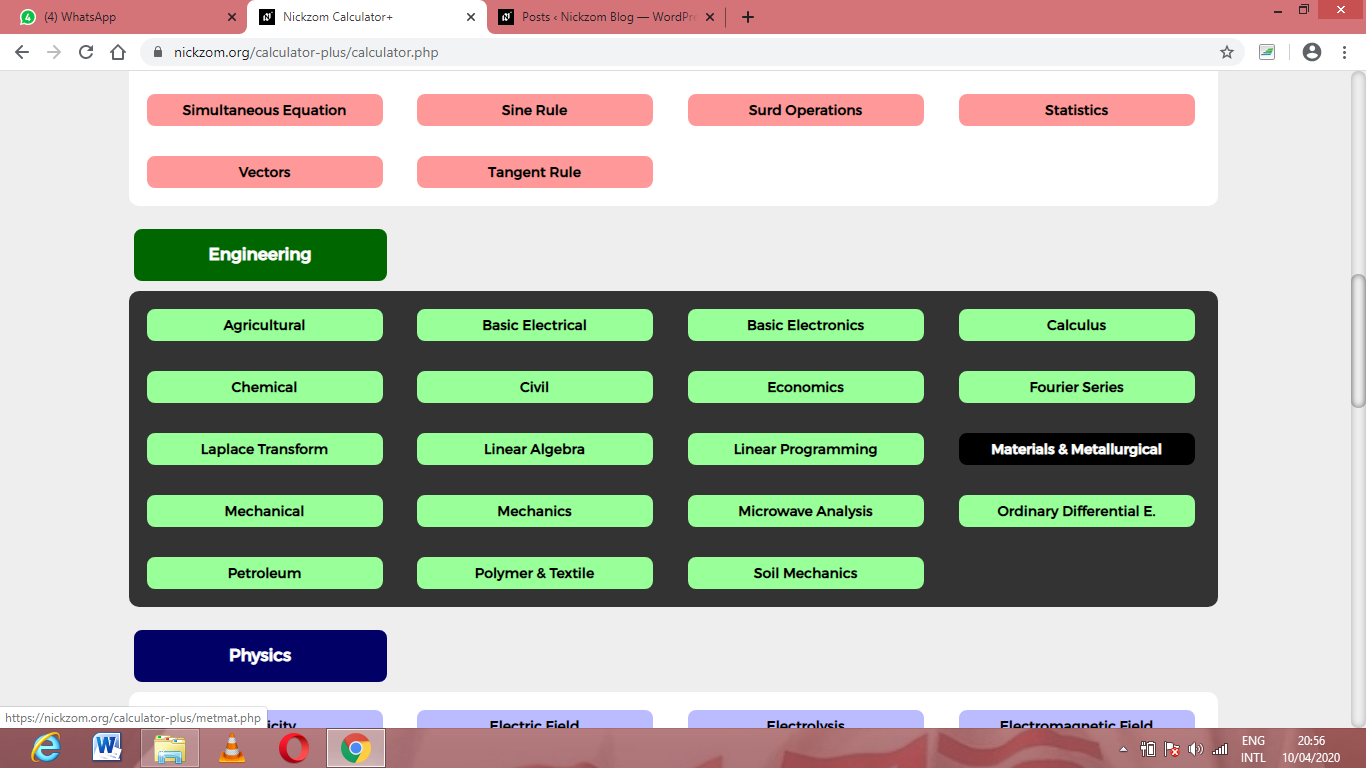Now, Click on Ceramics under Material & Metallurgical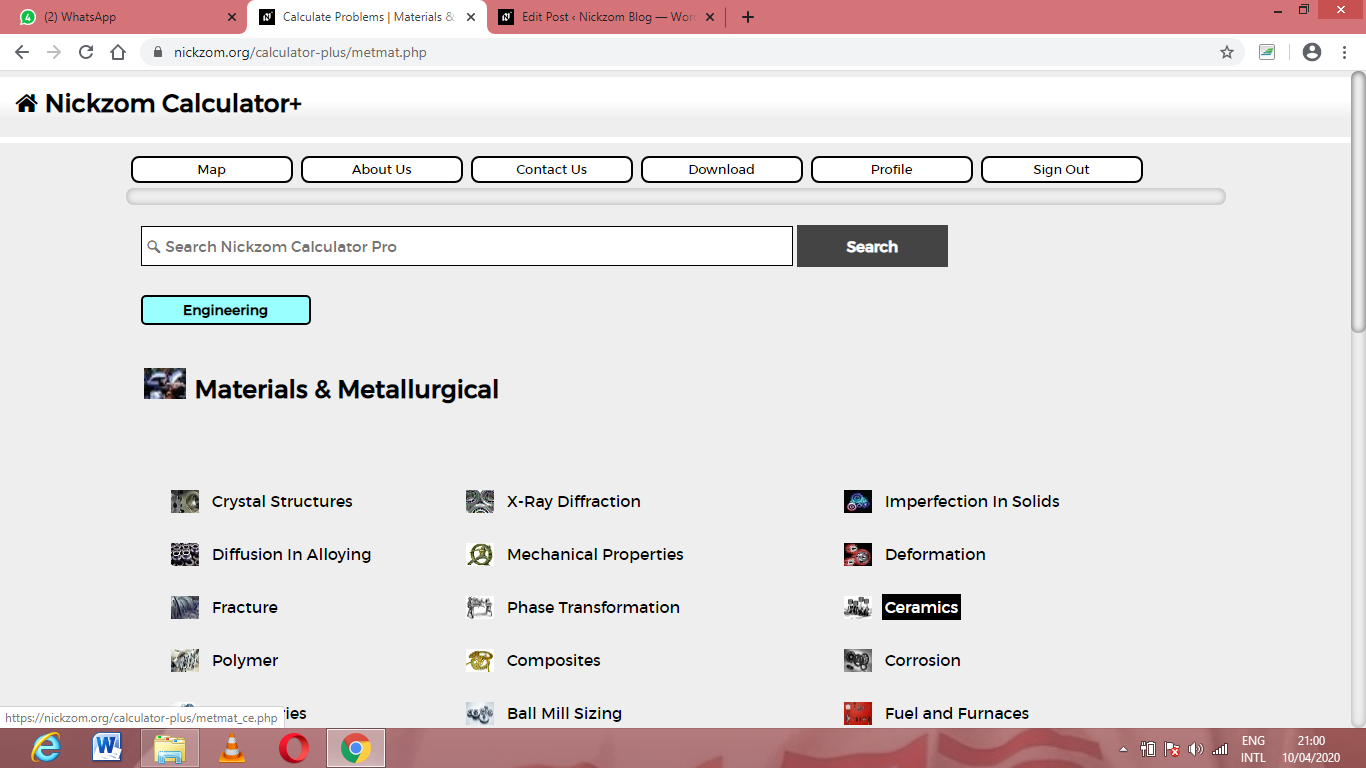Now, Click on Frenkel Defect under Ceramics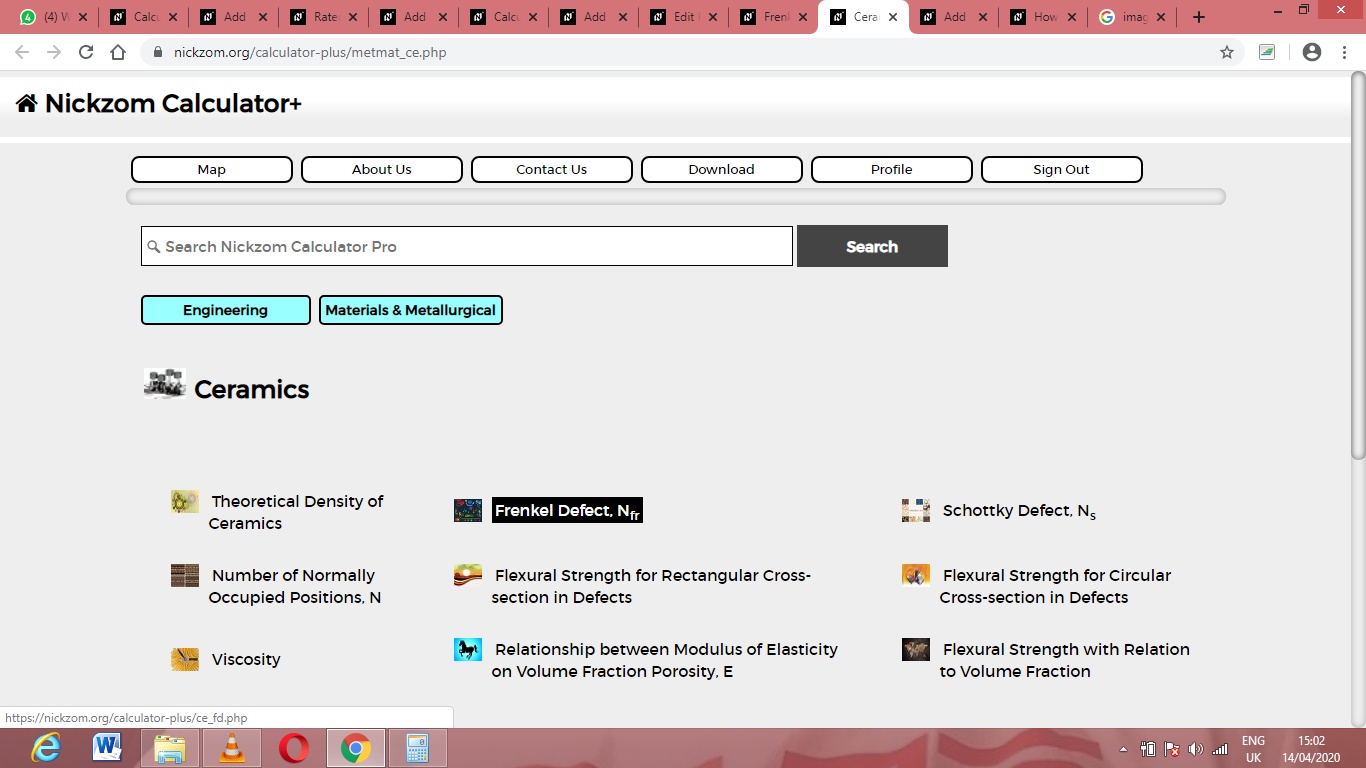The screenshot below displays the page or activity to enter your values, to get the answer for the frenkel defect according to the respective parameters which are the N, activation energy (Qfr), Boltzmann’s Constant (K) and temperature (T).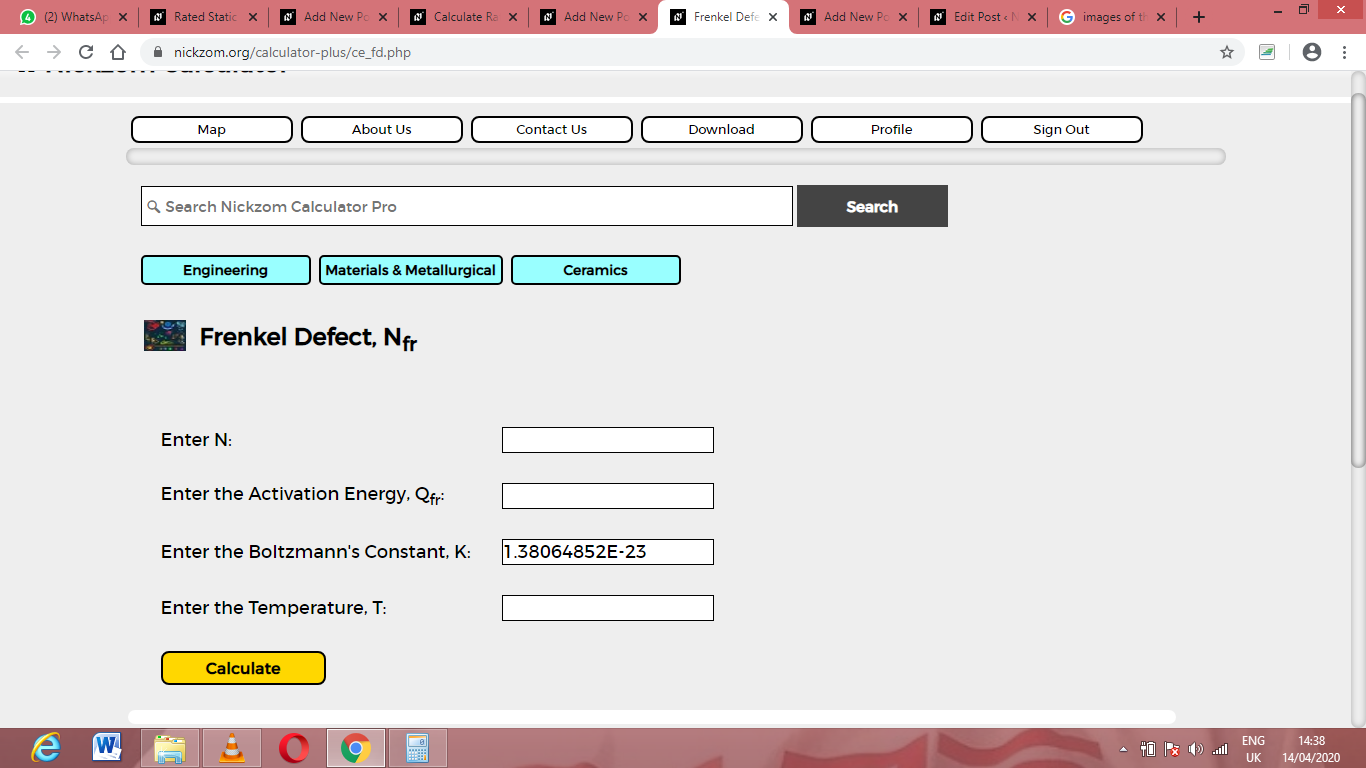Now, enter the values appropriately and accordingly for the parameters as required by the N is 22, activation energy (Qfr) is 34, Boltzmann’s Constant (K) is 1.38064852e-23 and temperature (T) is 12.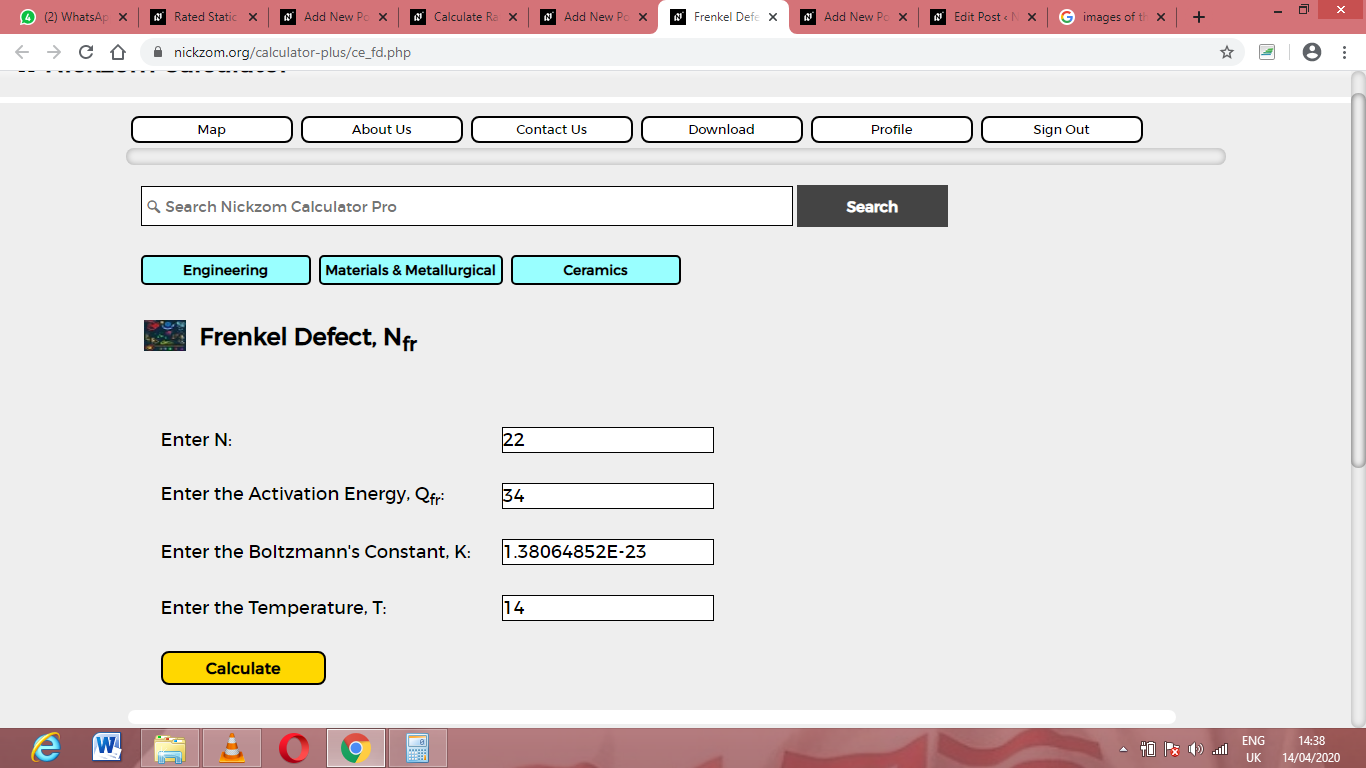Finally, Click on CalculateAs you can see from the screenshot above, Nickzom Calculator– The Calculator Encyclopedia solves for the frenkel defect and presents the formula, workings and steps too.